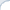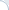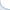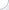## When average precision is equal to recall

In certain situations, an average precision is equal to recall. Proving that this is the case and finding ways to deal with this issue can be a good problem for an IR course homework and might help students to grok the notion of the average precision. This problem was inspired by the real research paper where authors used the mean average precision without realizing it was equal to recall.

Imagine that a nearest-neighbor search should retrieve 10 images most similar to a query image. The search is typically not ideal and some found images would be quite different from the query image. If we make humans judge retrieved results, it can be possible to evaluate search effectiveness via the (mean) average precision. If human judgments are not available, one may decide to use a proxy metric: An image is relevant if it is a true nearest neighbor, i.e., it is one of the 10 most closest images with respect to the values of the distance function. An exact search method always returns the true 10 nearest neighbors, but an approximate method may fail to do so. A degree of "sloppiness" can be estimated using the average precision, right?

I think that this wrong and here is why. In this formulation, a retrieved image is relevant when a distance from this image to the query object does not exceed the distance from the query image to the 10-th true nearest neighbor. Furthermore, all returned objects are normally sorted in the order of increasing distance from the query. Consequently, there will be $R\le10$ objects with distances not exceeding the distance to the 10-th nearest neighbor. And these images will clearly be considered "relevant". The following $10-R$ images will have distances larger than the distance to the 10-th nearest image and, thus, they will not be considered relevant.

It is not hard to see that in this case the average precision is equal to $R/10$ (by the definition of the average precision, P@N is one if $N \le R$ and is zero otherwise). However, the recall (the fraction of true nearest neighbors returned) is also $R/10$.

A good homework problem can ask the following:

1) To prove the equality of the average precision and the recall;
2) To describe ways of dealing with this issue, i.e., can we still use the average precision (after some fixes)? If not, what else can be used?

The second question is clearly open-ended, because (in addition to introducing real human judgments) multiple solutions are possible, which include but are not limited to using a rank approximation error.

## Neat code to compare floating-point numbers

Floating point arithmetic is not exact and not even deterministic. Not only results may be different across platforms and compilers, but in a non-strict (but fast) mode an outcome of the same operation may depend on the invocation context. If you use an aggressively optimizing compiler (such as Intel), it is silly to assume that same function arguments will always produce same results. The outcomes will be the same in many cases, but the results might also fluctuate by a few units in the last place (ULP). Code written with the assumption that results are always the same (i.e., assuming that floating point arithmetic is always consistent) may contain bugs that are hard to reproduce and fix.

To avoid such bugs, we may need to compare floating points numbers approximate rather than exactly. So, how do we do this? It is apparently easy to come up with a simple comparison code that works in most cases, but likely to fail in some rare cases. After some googling, I found a neat implementation of the Bruce Dawson algorithm from "Comparing Floating Point Numbers". The implementation was buried in the Google C++ Test Framework (authors Zhanyong Wan and Sean Mcafee). Thanks to Fred Richards, it was extracted and repackaged (see Fred's blog entry for a detailed description of the algorithm).

Fred's version was a bit inflexible and included only the hard coded threshold value (for numbers to to be considered the same). Thus, I had slightly modified the code to accept a threshold value as an argument of the comparing function. In addition, I made the ULP_diff function publicly available and improved the testing example. If you need a code to compare floating point numbers approximately, you can grab it from my repository.

UPDATE: I forgot to mention that Boost does have the code with similar functionality. Yet, I was looking for the code without Boost dependencies.

## What's wrong with _mm_extract_ps and _mm_extract_pd?

If you are programming in C/C++, you can use Single-Instruction Multiple Data(SIMD) commands. The beauty of these commands is that they operate on small vectors rather than singular scalar values. For example, we can multiply or subtract 4 pairs of floating point numbers at the same time. SIMD commands are available on many CPUs, but my post is Intel-specific.

The current standard set of instructions on Intel SSE 4 supports vector operations on 128-bit data elements. The vector size in bits is always 128, but the size of data elements is different. You can operate on sixteen 8-bit values, eight 16-bit values, four 32-bit values, and two 64-bit values. The type of data elements can also be different. A 32-bit value, e.g., can be an integer or a single-precision floating point number. A 64-bit value can be an integer, or a double-precision floating value. Longer, e.g., 256-bit vectors are also supported by newer CPUs, but I do not consider them here.

And it is kinda nightmarish, because you cannot use your regular '+', '-', '*' any more. You have a separate function for each data type (and, even worse, a bunch of conversion functions). For instance, addition of two 4-element floating point vectors is _mm_add_ps, addition of two 2-element double-precision floating point vectors is _mm_add_pd, and addition of two 4-element integer vectors is _mm_add_epi32

It is bad, but not terribly bad, because there is a naming convention that helps you navigate through this jungle. As you might have noticed, all operations start with the same prefix _mm_, then there is a part indicating the type of the operation, and, finally, a type-specific suffix. These suffixes are as follows:

epi8 for 8-bit integers;
epi16 for 16-bit integers;
epi32 for 32-bit integers;
ps for single-precision floating point numbers;
pd for double-precision floating point numbers.

To operate on 128-bit vectors, the CPU uses special 128-bit registers. If you need to extract specific vector elements and store them in regular 32-bit or 64-bit registers, you have to use a special CPU command. Of course, you can always copy vector values to the memory and read back only a necessary portion, but this is rather slow. This is why there are commands that can copy specific elements of a 128-bit vector to a 32-bit or 64-bit CPU register. BTW, store and load operations also follow a convention. The store command for four-element single-precision floating point vectors is _mm_storeu_ps (u in storeu denotes an unaligned write).

The command _mm_exctract_epi8 treats a 128-bit register as a 16-element integer vector. It allows one to extract any of the sixteen integer vector elements (each has a size of 8 bit). _mm_extract_epi16 gives you one of the eight 16-bit vector elements_mm_extract_epi32 extracts one of the four 32-bit integer values. Ok, what does _mm_extract_ps do? Extracts one of the four single-precision floating point numbers, right? Wrong, it also extracts one of the four 32-bit integers. Furthermore, there is no function _mm_extract_pd!

To efficiently extract floating point numbers you need to use functions _mm_cvtss_f32 and _mm_cvtsd_f64. They extract only the first floating point number from the vector. Yet, there is a command to move an arbitrary element of the four-element vector to the first position. This command is called a shuffle instruction. Thus, you can first shuffle an arbitrary element to the first position, and then extract the first element. The name of the shuffle command is a bit of misnomer itself, because shuffle usually means rearranging. Yet, shuffling on Intel is IMHO multiplexing.

It does not bother me much that the actual floating-point extraction functions are missing. Yet, I cannot understand why there is a function _mm_extract_ps with a misleading name and redundant functionality? Anyway, after reading some material on the Web, I have created two simple macros: one for extraction of single-precision and and another for extraction of double-precision floating point numbers. My code is freely available.

## How fast is UIMA subiterator function?

Assume that you need to identify part of speech tags (POS-tags) in a sentence: "My dog likes meat". You run a POS-tagger and get the following result (pronouns are green, nouns are blue, and verbs are red):

My dog likes meat

One can view such process as highlighting words using pens of different colors. We do not change the original text, just add additional information. Computerized processing of natural languages adopted this paper-and-pen model by introducing a concept of electronic annotations. An annotation is simply a record that "highlights" a span of characters. The annotation has a start, an end, a type, and a number of attributes. In our example, all annotations are of the same type, but their color attribute allows us to distinguish among different POS tags. In an NLP world everything is annotation!

Annotations are typically created by different annotation tools independently. Yet, we frequently need to know which annotations overlap. For example, sentence boundaries may be denoted by annotation of one type. Tokens and POS tags could be annotations of a different type. Figuring out which POS tags are assigned to tokens in a given sentence requires us to identify POS tags and tokens that overlap with the sentence (or rather tokens/tags that are contained in it) .

Probably, you do not want to invent a wheel and implement annotation processing using some off-the-shelf software. In particular, our group employs UIMA ECD, a framework on top of Apache UIMA. (For more details on UIMA-ECD, please, see a tutorial. BTW, UIMA-ECD is much easier beast to tame than pure UIMA). An overlap of annotations is computed using the function subiterator. (see also my recent comment on type priorities.)

How efficient is subiterator? In theory, UIMA uses indexes, but I could not find any comments on the efficiency of this operation in the official documentation. I tried to look at the source code, but it was hard for me to get through the maze of classes and abstract interfaces quickly. Anyways, even if UIMA uses some form of an index, how efficient is such an index? Being a bit paranoid, I decided to benchmark.

To this end, I created a simple pipeline. In this pipeline, I removed HTML from Wikipedia documents. Then, documents were annotated using the SENNA parser and OpenNLP. OpenNLP creates annotations for sentence boundaries, while the SENNA parser identifies POS tags (recall that sentence boundaries are denoted by annotations).

Finally, I iterated over sentences and for each sentence I retrieved related POS tags using two approaches. The first approach employed subiterator and was expected to be efficient (due to relying on indexes). In the second approach, I simply iterated over all POS tags in a document. This one would be slow, because a Wikipedia document has thousands of POS tags and hundreds of sentences. Thus, a nested loop (first over sentences, then over POS tags) could be expensive. My code is freely available.

Depending on a document, an average time to retrieve a POS tag using an index varied from 1 to 5 microseconds. In the bruteforce-iteration approach, which does not rely on index, a time to retrieve a POS tag varied from 0.1 to 0.5 millisecond, a two orders of magnitude difference.

Conclusions? A UIMA subiterator function is not terribly fast (1-15K CPU cycles per operation), but it is rather efficient. I would say it should be good enough for most tasks.

UPDATE1: This example will always work if a sentence span is strictly larger than the span of a contained annotation. If the spans can be of equal size, one needs to properly define type priorities. In that, the Sentence will need to have a higher priority.

UPDATE2: See also a follow-up post. There is a more efficient UIMAfit implementation of the subiterator function that also does not care about type priorities.

## Pages